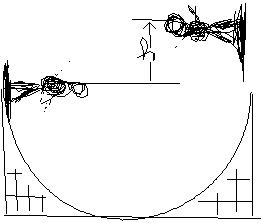Question# FSP02510402421.jpgFSZThe skateboarder in the drawing starts down the

Other
ANSWEREDThe skateboarder in the drawing starts down the left side fthe ramp with an initial speed of 5.4 m/s. If nonconservativeforces, such as kinetic friction and air resistance, arenegligible, what would be the height h of the highestpoint reached by the skateboarder on the right side of the ramp?$$\displaystyle{2}={{v}_{{0}}^{{2}}}+{2}{a}{d}$$
(where v=final velocity, $$\displaystyle{v}_{{0}}=$$ starting velocity, a=acceleration,d= displacement)
$$\displaystyle{0}\frac{{m}}{{s}}={\left({5.4}\frac{{m}}{{s}^{{2}}}\right)}+{2}{\left(-{9.8}\frac{{m}}{{s}^{{2}}}\right)}{d}-{29.16}\frac{{m}}{{s}^{{2}}}=-{19.6}\frac{{m}}{{s}^{{2}}}$$